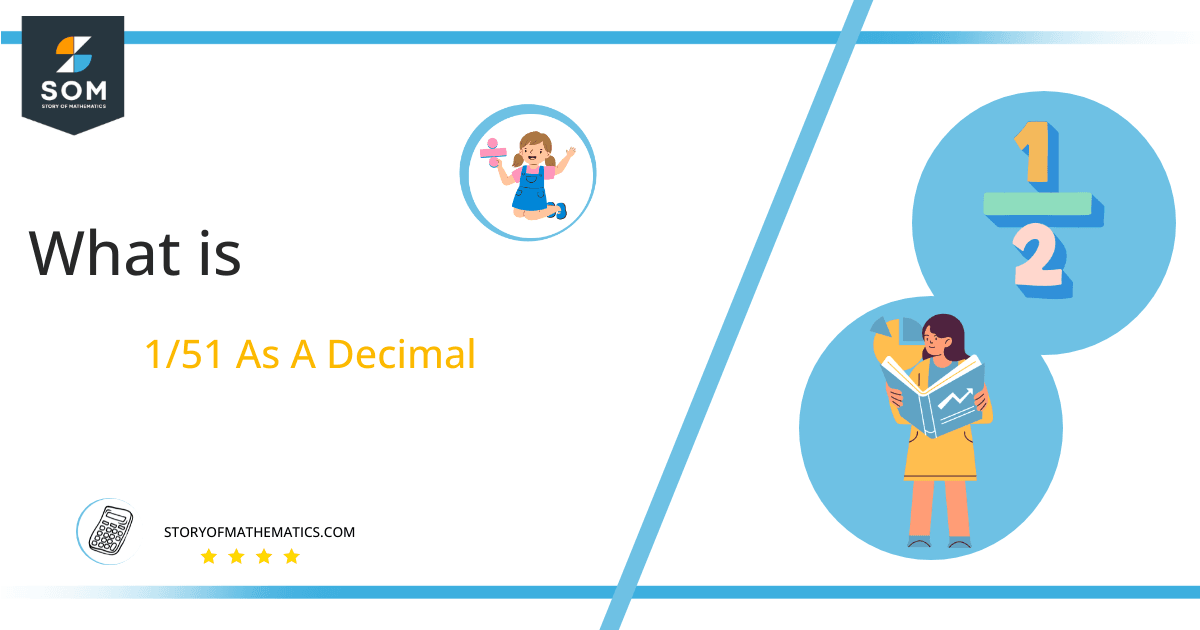# What Is 1/51 as a Decimal + Solution With Free Steps

The fraction 1/51 as a decimal is equal to 0.019.

A mixed fraction is the simplified version of the improper fraction. It consists of an integer number that is taken from the improper fraction and the remainder value is depicted by a proper fraction coupled together.Here, we are more interested in the division types that result in a Decimal value, as this can be expressed as a Fraction. We see fractions as a way of showing two numbers having the operation of Division between them that result in a value that lies between two Integers.

Now, we introduce the method used to solve said fraction to decimal conversion, called Long Division, which we will discuss in detail moving forward. So, let’s go through the Solution of fraction 1/51.

## Solution

First, we convert the fraction components, i.e., the numerator and the denominator, and transform them into the division constituents, i.e., the Dividend and the Divisor, respectively.

This can be done as follows:

Dividend = 1

Divisor = 51

Now, we introduce the most important quantity in our division process: the Quotient. The value represents the Solution to our division and can be expressed as having the following relationship with the Division constituents:

Quotient = Dividend $\div$ Divisor = 1 $\div$ 51

This is when we go through the Long Division solution to our problem. Given is the long division process in Figure 1:Figure 1

## 1/51 Long Division Method

We start solving a problem using the Long Division Method by first taking apart the division’s components and comparing them. As we have 1 and 51, we can see how 1 is Smaller than 51, and to solve this division, we require that 1 be Bigger than 51.

This is done by multiplying the dividend by 10 and checking whether it is bigger than the divisor or not. If so, we calculate the Multiple of the divisor closest to the dividend and subtract it from the Dividend. This produces the Remainder, which we then use as the dividend later.

Now, we begin solving for our dividend 1, which after getting multiplied by 10 becomes 10.

We take this 10 and divide it by 51; this can be done as follows:

10 $\div$ 51 $\approx$ 0

Where:

51 x 0 = 0

This will lead to the generation of a Remainder equal to 10 – 0 = 10. Now this means we have to repeat the process by Converting the 10 into 100 and solving for that:

100 $\div$ 51 $\approx$ 1

Where:

51 x 1 = 51

This, therefore, produces another Remainder which is equal to 100 – 51 = 49. Now we must solve this problem to Third Decimal Place for accuracy, so we repeat the process with dividend 490.

490 $\div$ 51 $\approx$ 9

Where:

51 x 9 = 459

Finally, we have a Quotient generated after combining the three pieces of it as 0.019, with a Remainder equal to 31.Images/mathematical drawings are created with GeoGebra.